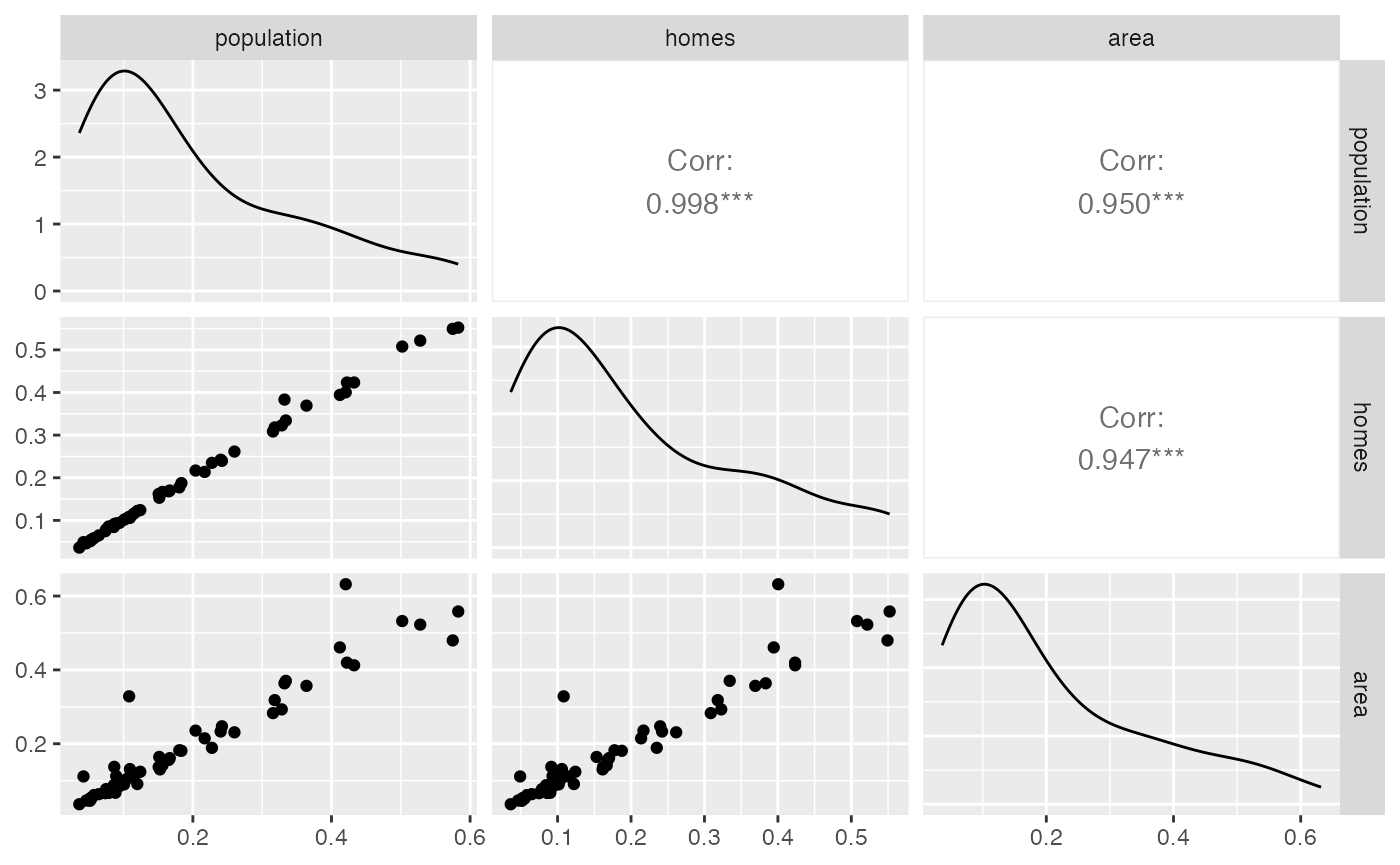Spatial interpolation is the process of transforming data from one spatial resolution to another (e.g. from census tract to ZIP code). The cincy::interpolate function uses census block-level weights to interpolate data from one cincy geography to another cincy geography. Variables prefixed with n_ will be interpolated extensively (weighted sum), and all other numeric variables will be interpolated intensively (weighted mean).

Here, we interpolate fraction_poverty from the 2018 American Community Survey from 2010 census tracts to 2010 Neighborhoods and 2010 ZCTAs.

library(cincy)
library(dplyr)
library(sf)
library(tmap)
library(tidyr)

d_tract <- st_transform(cincy::dep_index, 3735) |>
select(census_tract_id_2010, fraction_poverty)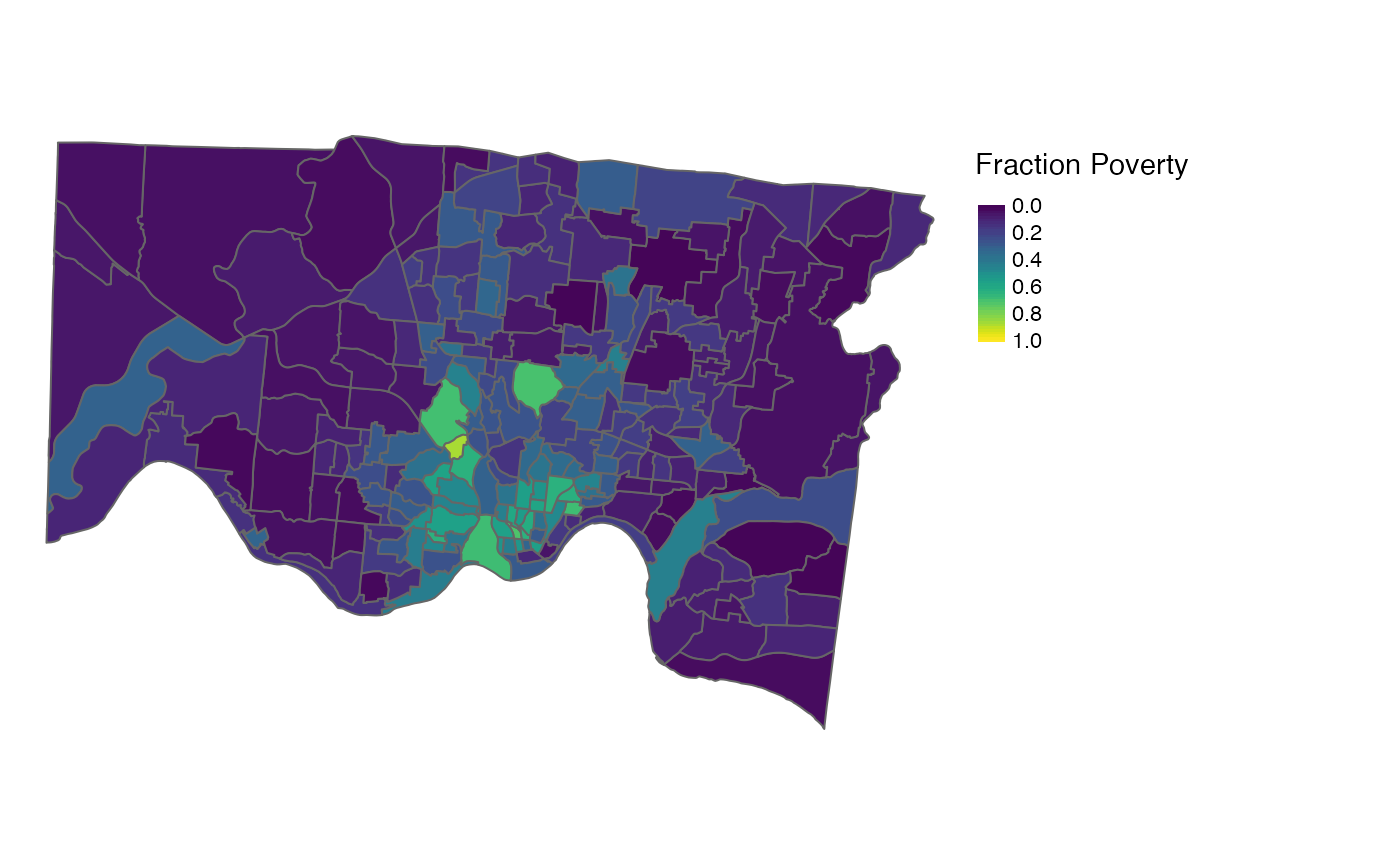## Interpolating to Neighborhood and ZCTA

The cincy::interpolate function allows the user to choose from three sets of weights to be used for the weighted sums and/or averages: block group population, block group number of homes, and block group area.

Neighborhood

d_neigh_pop <-
d_tract |>
cincy::interpolate(to = cincy::neigh_cchmc_2010, weights = "pop") |>
st_transform(3735)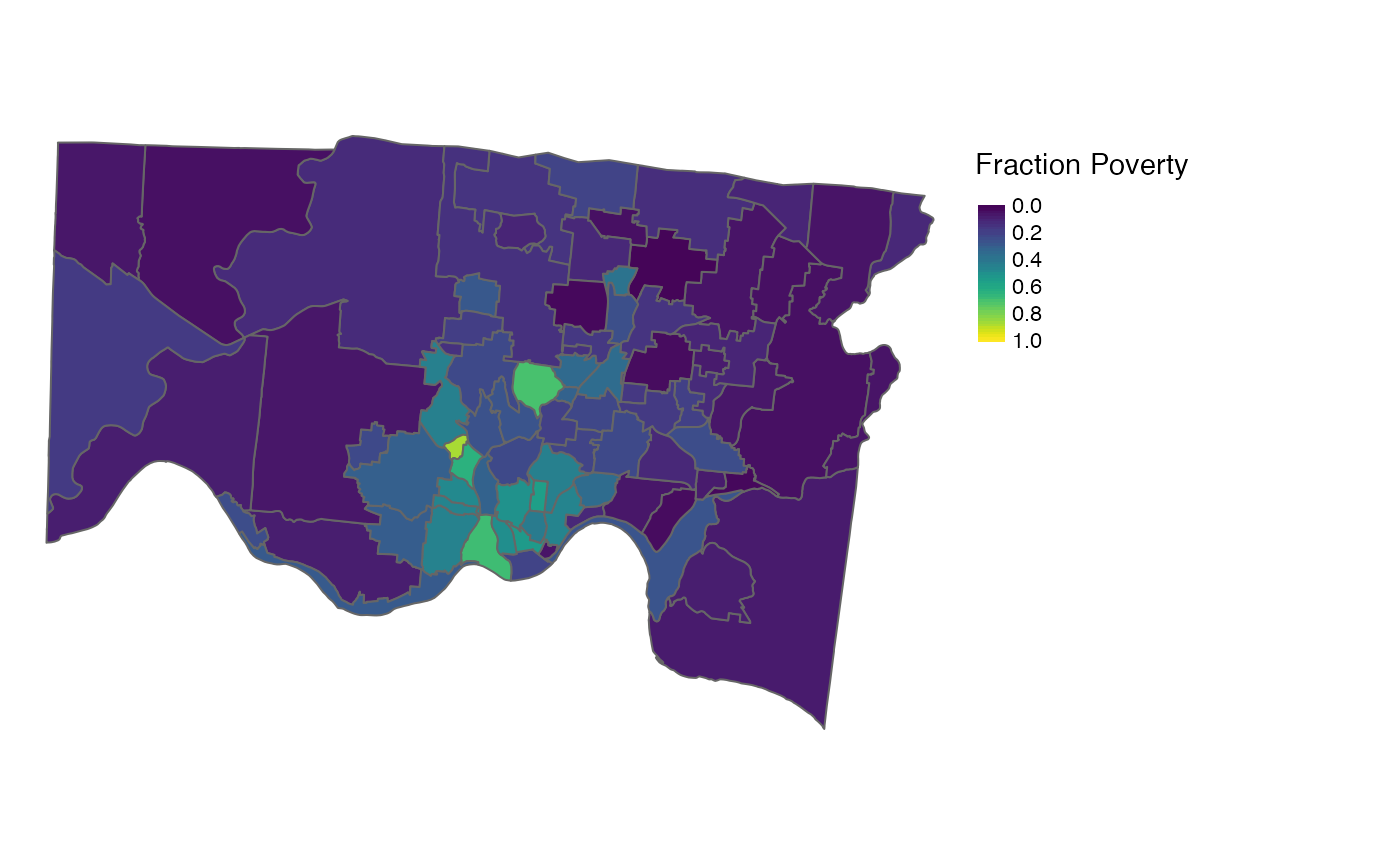ZCTA

d_zcta_pop <-
d_tract |>
cincy::interpolate(to = cincy::zcta_tigris_2010, weights = "pop") |>
st_transform(3735)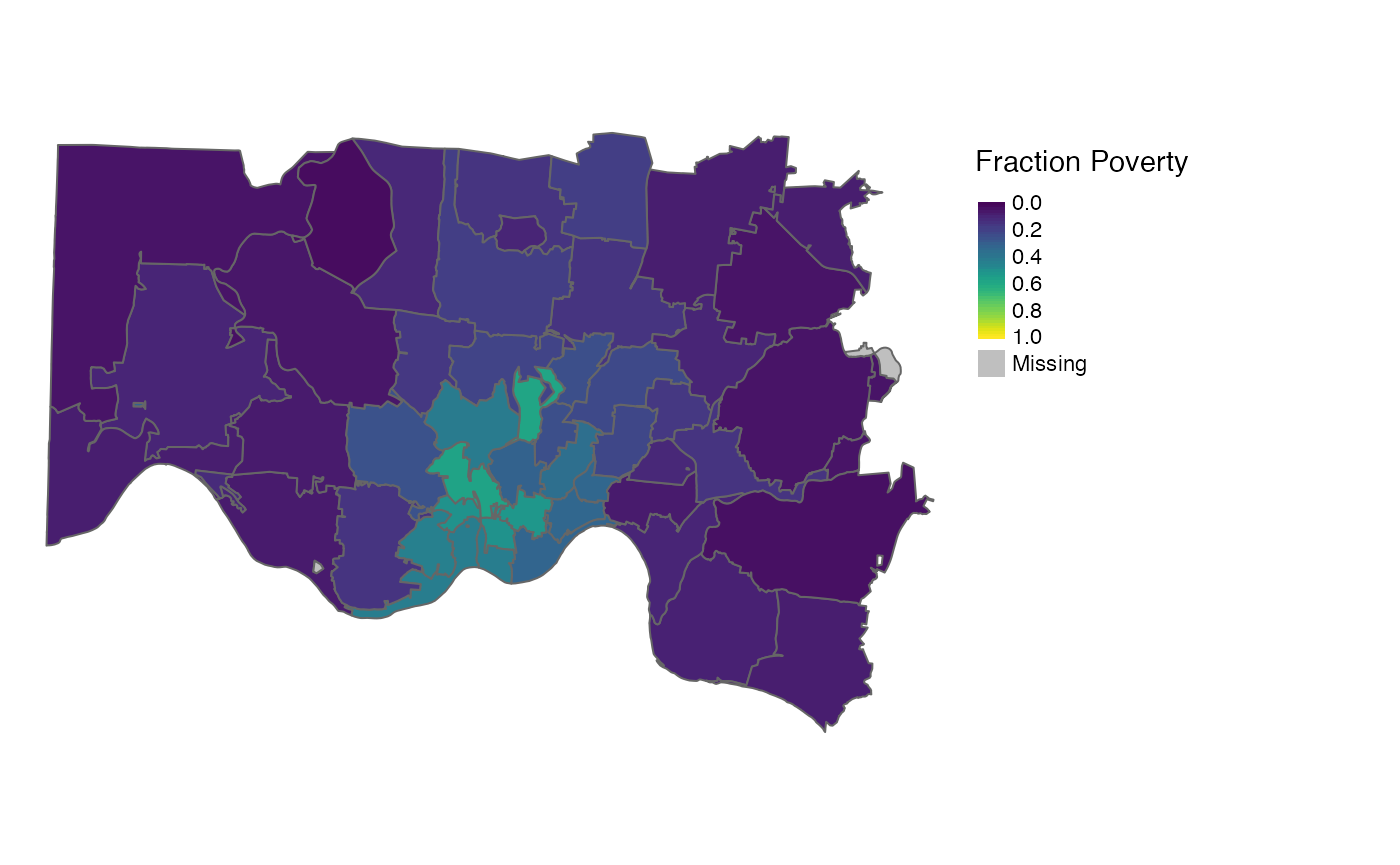The above examples use population (pop) weights, but homes or area weights can be used by changing the weights argument.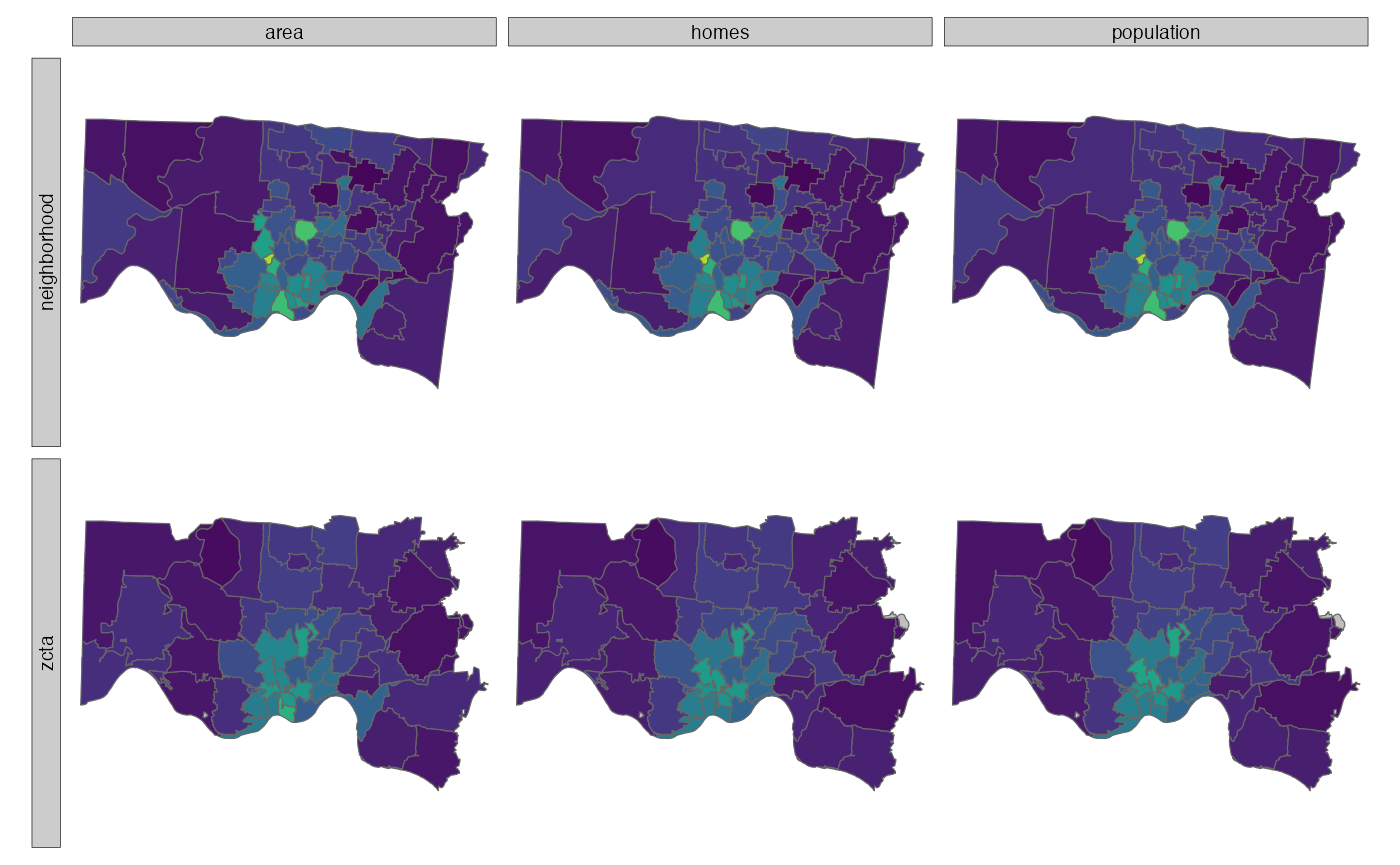Note that we lose variability when interpolating from a smaller geography to a larger geography. This is especially noticeable when interpolating from tract to ZCTA.

Also note that all three weights yield similar results in this example. However, the result of using area weights varies slightly compared to homes and population. This conclusion is further supported by the correlation between interpolated fraction_poverty values using the three sets of weights.

Neighborhood fraction_poverty interpolated from tract using population, homes, and area weights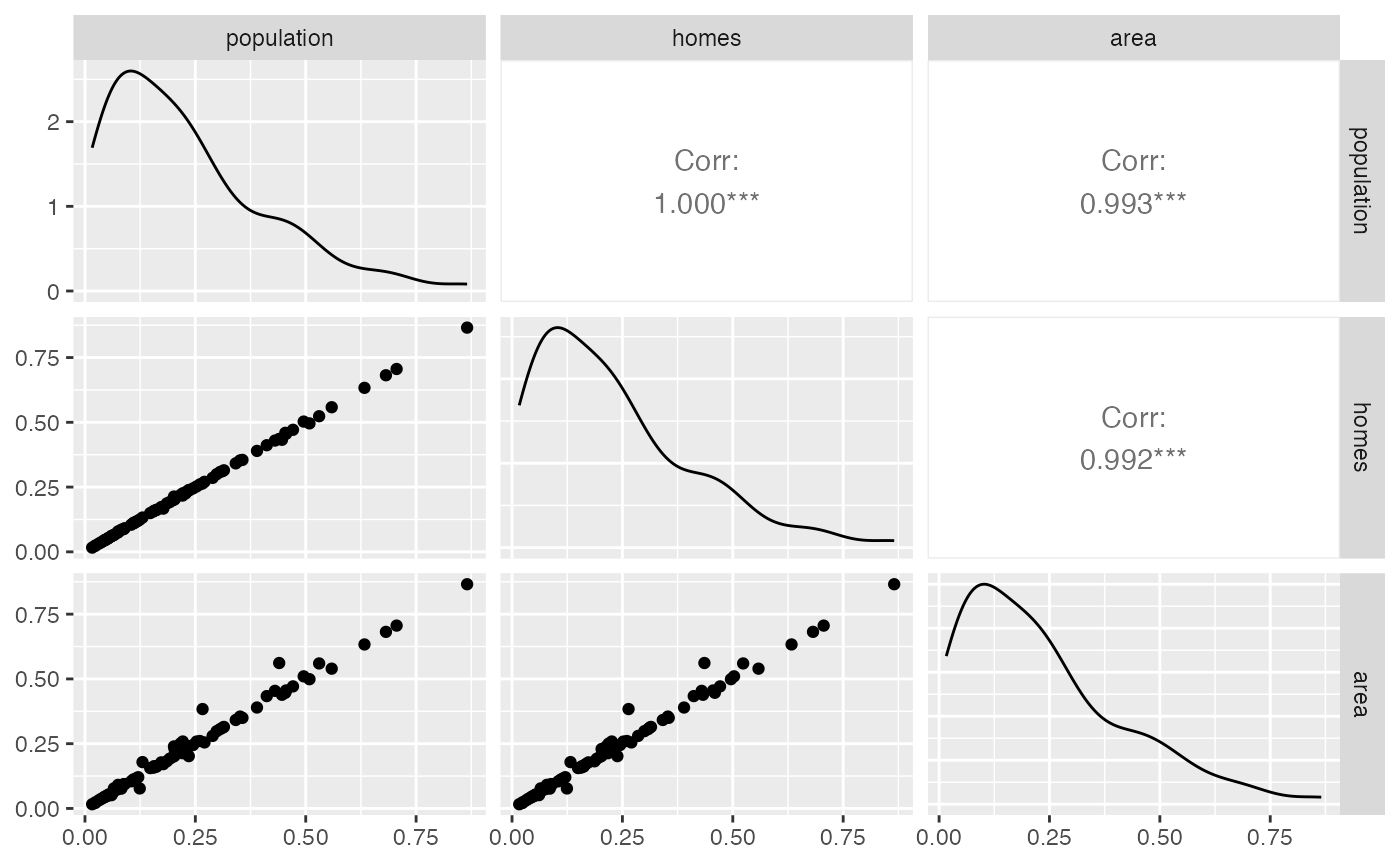ZCTA fraction_poverty interpolated from tract using population, homes, and area weights